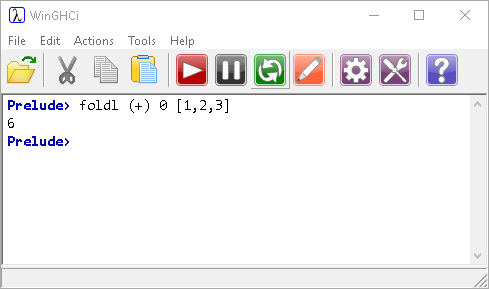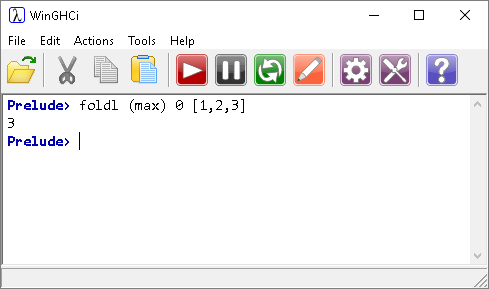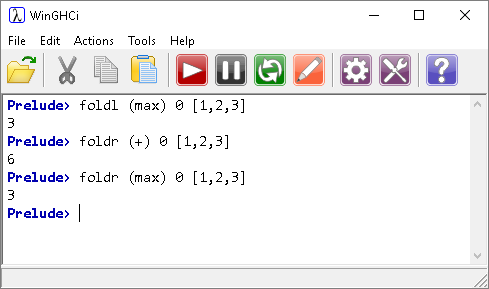## Introduction

The two functions foldl and foldr are used to apply a combining function to a list.

The two functions differ in the direction in which they are applied to the list. The foldl functon works from the left of the list, the foldr, works from the right of the list.

## Left Fold - foldl

Study this example,The list [1,2,3] is added to the accumulator (0), one item at a time. Each time an element is added to the accumulator it is added to the list, it is removed from the list. This continues until there are no items in the list.

The function used (+) is a binary function, it takes two operands. The accumulator and an item from the list are the operands.

The left fold is so called because it works from the left side of the list. In the above example, direction is irrelevant.

We can use a function like max, to find the largest item in a list. The max function normally takes two parameters. The following repeatedly applies the max function to the accumulator and successive values in the list until the whole list has been processed. After the first list item is folded, the accumulator will always store the largest value so far.## Right Fold - foldr

The right fold works just the same as the left one. The only difference is that it works from right to left instead. The examples used for foldl all work in both directions,